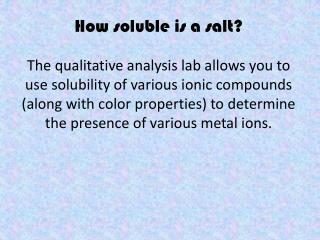# How soluble is a salt? - PowerPoint PPT PresentationDownload PresentationHow soluble is a salt?

How soluble is a salt?Download Presentation## How soluble is a salt?

- - - - - - - - - - - - - - - - - - - - - - - - - - - E N D - - - - - - - - - - - - - - - - - - - - - - - - - - -
##### Presentation Transcript

1. How soluble is a salt? The qualitative analysis lab allows you to use solubility of various ionic compounds (along with color properties) to determine the presence of various metal ions.

2. When we studied equilibrium, we used K as an indicator of ‘how far to the right’ a given reaction will proceed. For instance, in the following reaction H2 (g) + I2 (g) ⇄ 2 HI (g) K = 56 This means that at equilibrium, there is a higher concentration of HI than of H2 or I2. This is a product-favored reaction. Remember, K =

3. ….On the other hand 3 O2 ⇄ 2 O3 K = 6.25 x 10-58 Is a VERY reactant favored reaction. There is a little, teeny bit of O3 at equilibrium - not nearly as much as there is O2.

4. Then again we used K as an indicator of how strong acids and bases are. HCH3COO + H2O ⇄ CH3COO- + H3O+ Ka = 1.8 x 10-5 This is a reactant-favored reaction, and a pretty weak acid! The Ka here tells us what fraction of the HCH3COO will dissociate . H2CO3 + H2O ⇄ HCO3- + H3O+ Ka = 4.7 x 10-7 is an even weaker acid. Just look at it’s Ka value.

5. The relative solubility of salts can also be analyzed using their solubility product constants (Ksp). Silver bromide, for example, is a highly insoluble ionic compound. AgBr (s) ⇄ Ag+ (aq) + Br- (aq), Ksp = 3.3 x 10-13 3.3 x 10-13 = [Ag+] * [Br-]. This means that the if you add 1 gram of solid AgBr to a beaker of water, the resulting concentrations of dissolved silver and bromide ions will be LOW! To the naked eye, this salt will appear totally insoluble.

6. It also means that if you took a solution with Ag+ ions in it, for instance – a solution of highly soluble AgNO3, and added to it an aqueous solution of NaBr (another very soluble salt), the Ag+ ions and Br- ions would react together forming a solid white precipitate – AgBr!!

7. That chemical reaction might look like this… AgNO3(aq) + NaBr(aq)  NaNO3(aq) + AgBr(s) might be rewritten as a net ionic equation… Ag+ (aq) + Br- (aq)  AgBr (s) The Na+ and NO3- ions are just spectators (they begin aqueous and they end aqueous), so we omit them from the net ionic equaiton.

8. Another example of a net ionic equation… 2KI (aq) + Pb(NO3)2 (aq)  2KNO3 (aq) + PbI2 (s) Might be rewritten: Pb2+ (aq) + 2 I- (aq) PbI2 (s)

9. Look at the first step of the qualitative analysis. Which of the following salts has the lowest value for Ksp? PbCl2 FeCl3 AlCl3 CaCl2 CuCl2 How do you know?

10. ….Now Write net-ionic equations for EACH precipitation reaction in the qualitative analysis. (ie. each of the five metal ions precipitates out of solution as an insoluble ionic compound or colored ionic complex like Al(OH)4-. Write net-ionic equations for the formation of each of these)